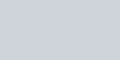# Lecture in Area Problem and the Riemann Sum Part 2

(Last Updated On: March 21, 2020)This is the part II of the series Area Problem and the Riemann Sum. It is important not to miss the part I before you continue on this topic.

### Riemann SumRiemann Sum is the total sum of n adjacent rectangles sideways on an interval [a,b] with a uniform positive widths and with heights determined by arbitrary values of a function.

### When is Riemann Sum have Positive values

• If f take positive values along the interval [a,b], just like in Figure 4, the function f is positive. All the heights of adjacent rectangles take positive values. In this case, we have adjacent rectangles with a positive heights and a positive widths. Therefore, The Riemann sum that approximates the area between the x-axis and f have positive values.
• A positive area represents the fact that the particular rectangle is above the x-axis.

### When is Riemann Sum have Negative values

• However, if f were to take some negative values along the interval [a,b], then some of the heights would be assigned negative values. Therefore, negative height values multiplied by negative width values give negative area values.
• A negative area represents that the rectangle is below the x-axis (since its height is given by a negative value of f ).

### What if function f takes both positive and negative values

• If f is continuous and takes positive and negative values along [a,b], then any corresponding Riemann sum approximates a net area where the net area equals the difference between the area of regions above the x-axis but under f and the area of regions below the x-axis but above f.
• In short, the areas of the rectangles above the x-axis minus the areas of the rectangles below the x-axis.

### Exact Net Area Between f and the x-axis

Until now, I know after all these things, you might be asking, How to get the exact area then?
Here is the idea, I suppose you have the knowledge about Limit. Using the Riemann sum approximation, we will define the exact net area between f and the x-axis as the limit of the sum of the areas of approximating rectangles as the number of rectangles approach infinity (n → ∞) .
Assume that f is a continuous function along the interval [a,b] . The net area A of the region bounded by f and the x-axis is given bywhere n represents the number of approximating rectangles with a uniform width equal to Δx = (b − a)/n and where xi represents some point in the ith subinterval of [a,b] .

### Definite Integral

As long as f is continuous the value of the limit is independent of the sample points xi used. The definite integral of f from a to b is the numberprovided the limit exists.

### Sample Riemann Sum Problems

1. Use a Riemann sum to estimate the area between f and the x-axis along the interval [1,3] forf (x) = 0.25x3 .
Answer: Using four rectangles and right end points as the sample point in each subinterval:2. Find the area between the curve y = x3 and the x-axis over the interval [0,2]. Use a sketches to show how to obtain over and under estimates for the area using Riemann sums.
Let y = f (x) . Note that the function increases. Arbitrarily select a number of rectangles like four. Determine the width of each rectangle.For an over-estimate, select heights in a manner that too much area is included. A right Riemann sum will do the trick as below.For an under-estimate, select heights in a manner that too little area is included. A left Riemann sum will suffice as shown.For your exercise selects heights in the midpoints, make the graph and find the area. Let me know how well you get the answer by providing feedback on the comment section.
The next topic is Finding Areas using Definite Integration. See you there.

credit: Randell Simpson (Temple College)©2014 www.PinoyBIX.com